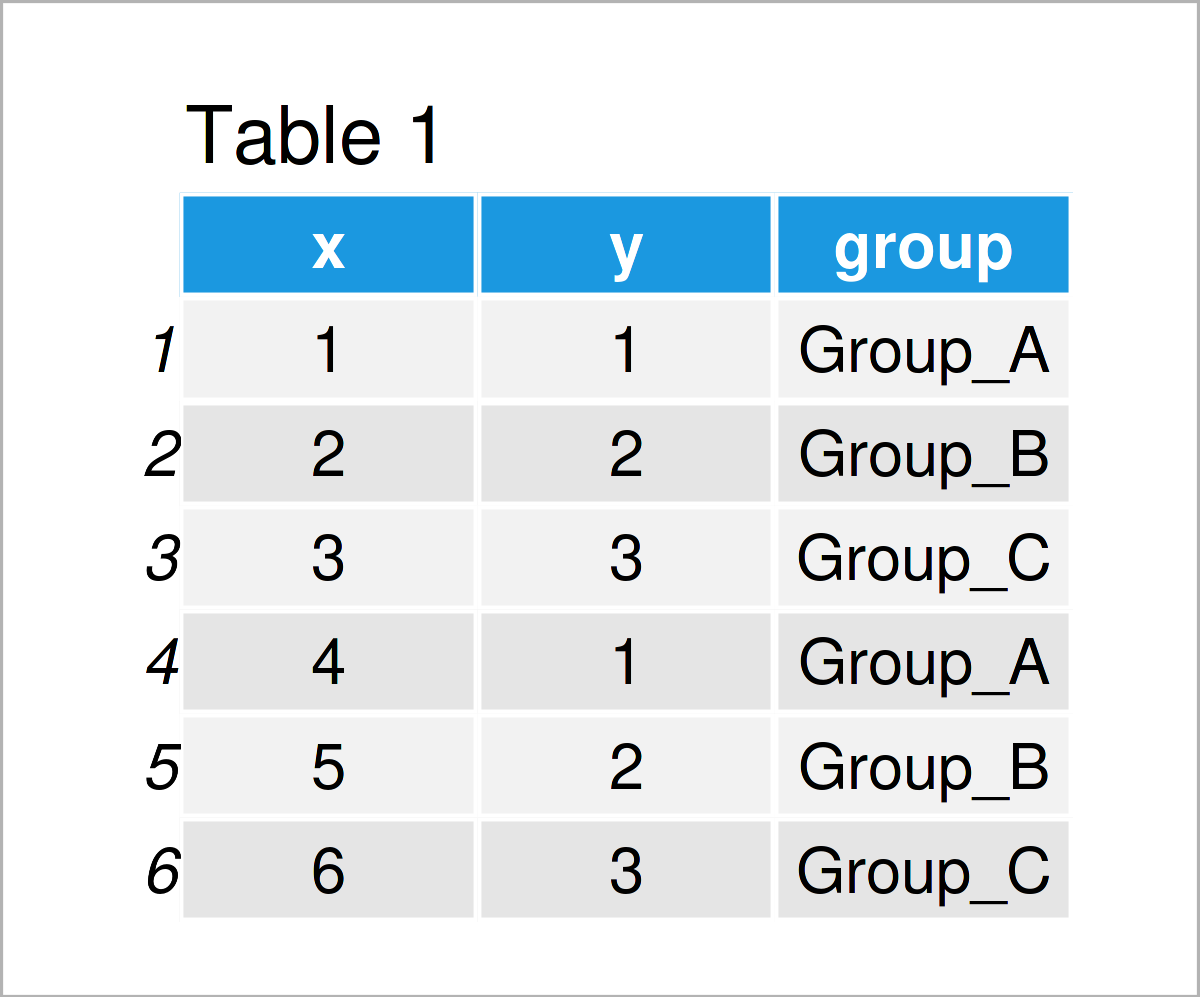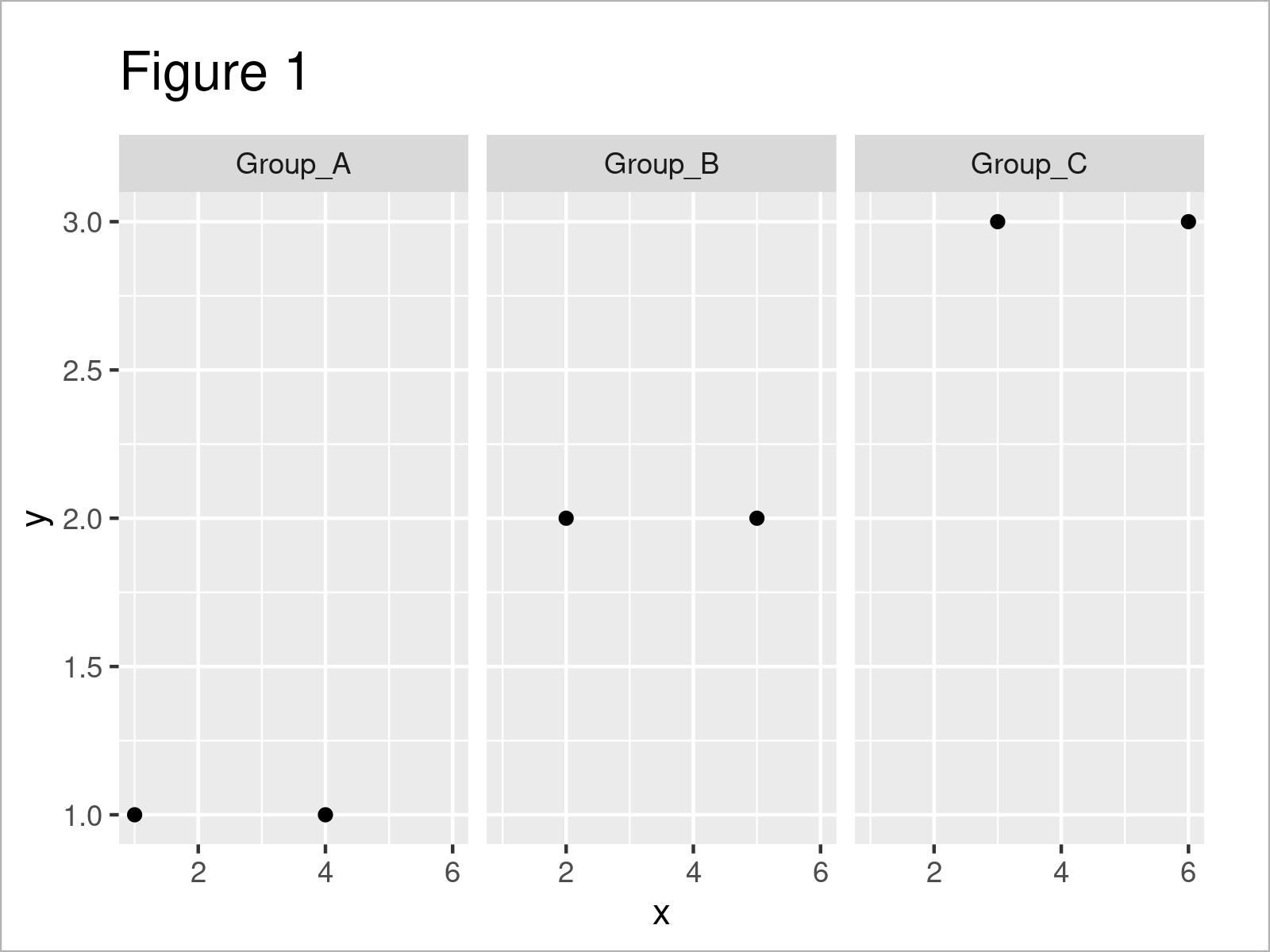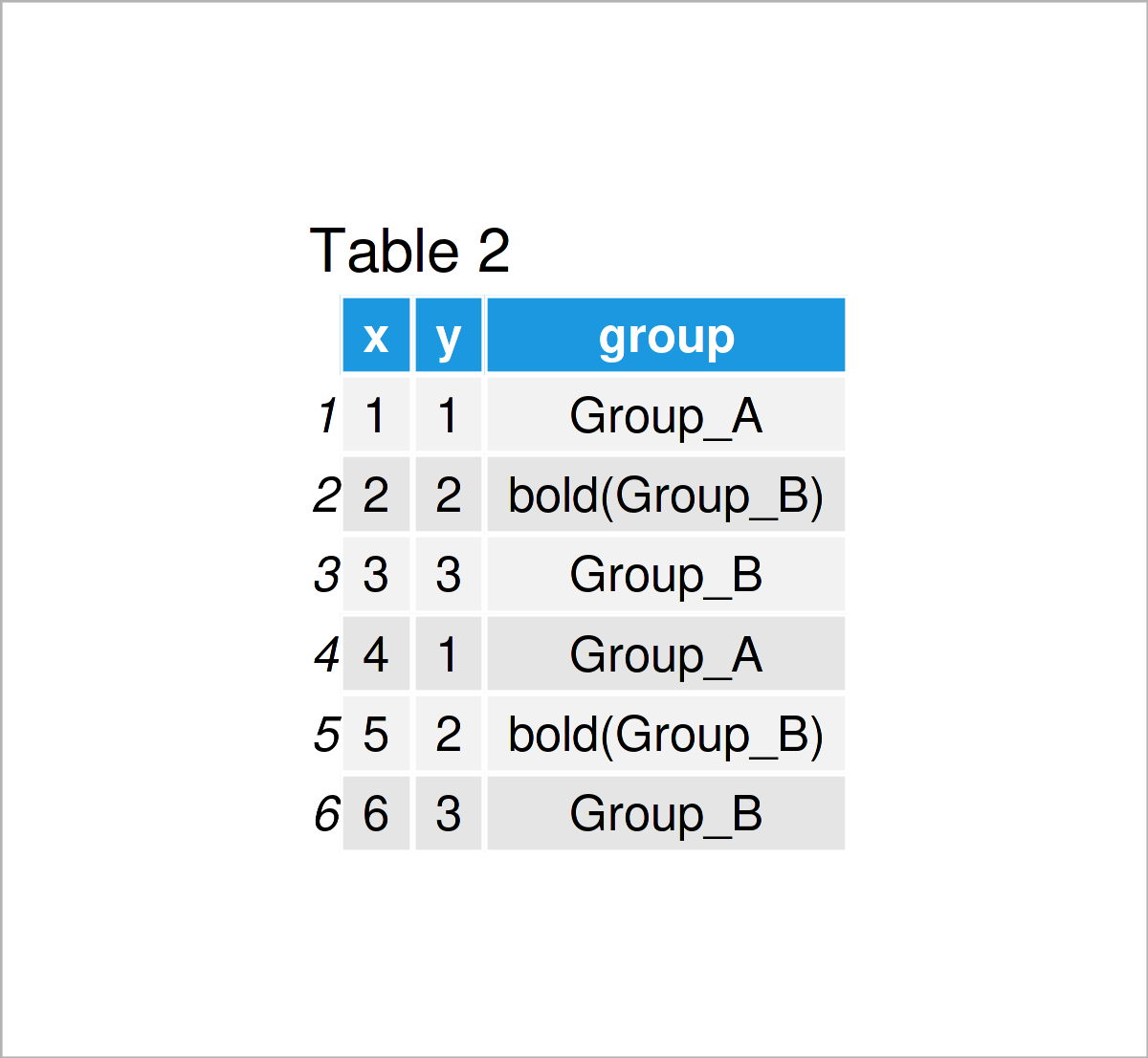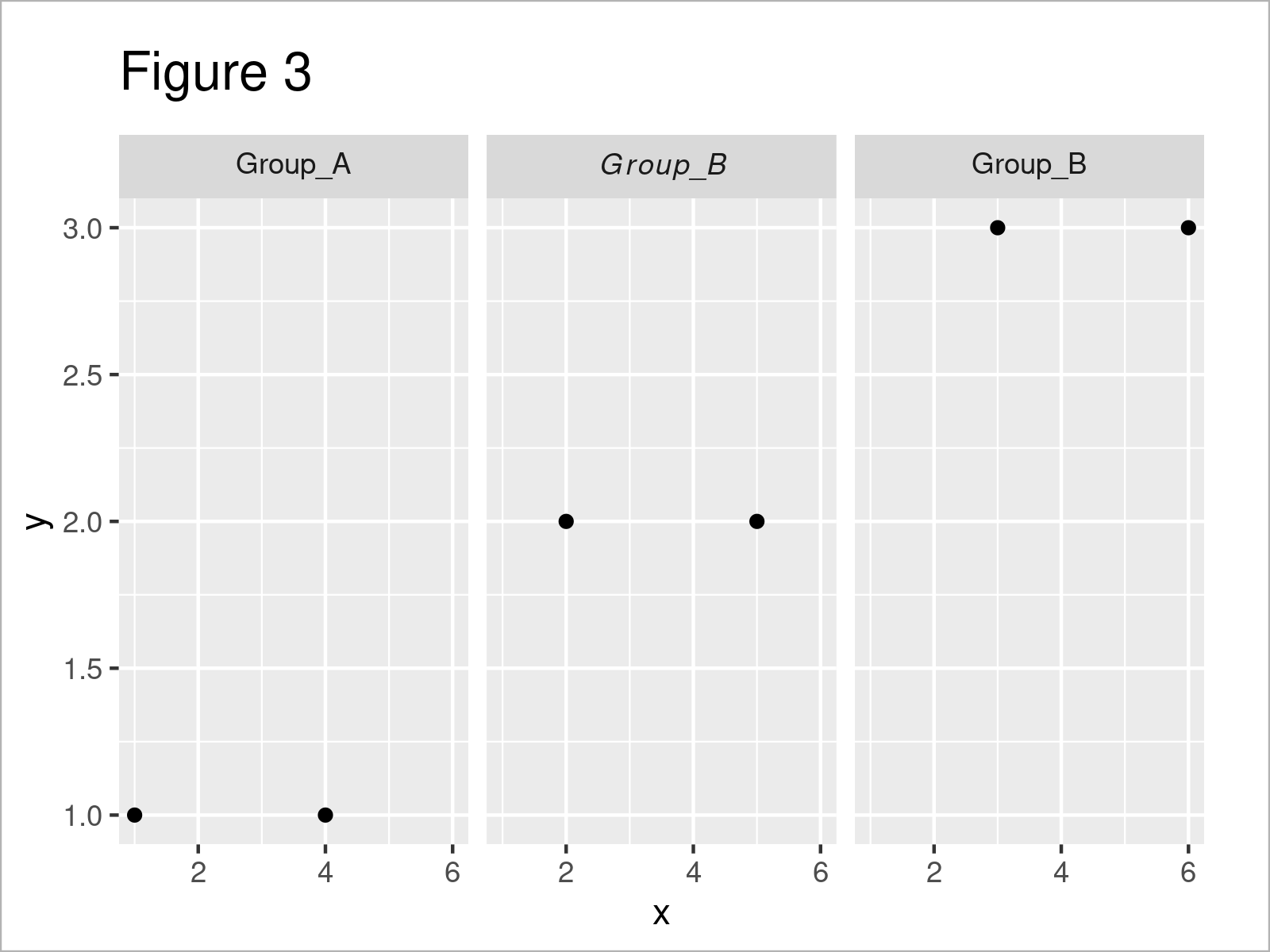# Change One Specific Label of ggplot2 Facet Plot to Bold or Italics in R (2 Examples)

In this R tutorial you’ll learn how to convert only one label of a ggplot2 facet plot to bold or italics.

The tutorial will contain the following content blocks:

Let’s get started!

## Example Data, Software Packages & Default Graph

We’ll use the following data as a basis for this R programming language tutorial:

```data <- data.frame(x = 1:6,                       # Create example data frame
y = 1:3,
group = paste0("Group_", LETTERS[1:3]))
data                                              # Print example data frame```Have a look at the previous table. It shows that our example data contains six rows and three columns.

If we want to draw a graphic of our data using the ggplot2 package, we also have to install and load ggplot2:

```install.packages("ggplot2")                       # Install & load ggplot2
library("ggplot2")```

As a next step, we can draw our data:

```ggplot(data, aes(x, y)) +                         # Draw ggplot2 facet plot
geom_point() +
facet_grid(. ~ group)```The output of the previous R programming code is visualized in Figure 1 – A ggplot2 facet plot with default labels.

Note that I’m using the facet_grid function in this tutorial. However, the same syntax could be applied to a ggplot2 facet plot created with the facet_wrap function.

Anyway, let’s move on to the examples!

## Example 1: Change One Specific ggplot2 Facet Plot Label to Bold

In this section, I’ll illustrate how to transform a certain label of a ggplot2 facet plot to a bold font style.

To accomplish this, we first have to change the factor labels of our grouping variable:

```data_bold <- data                                 # Duplicate data frame
data_bold\$group <- factor(data_bold\$group,        # Change factor labels
labels = c("Group_A",
"bold(Group_B)",
"Group_B"))
data_bold                                         # Print updated data frame```As shown in Table 2, we have created a new data frame where the labels of the column group have been changed. As you can see, we have specified “bold()” for each row of Group_B.

Now, we can use these data to modify only the second label of our facet plot. Note that we also have to use the labeller argument within the facet_grid function to parse our factor labels:

```ggplot(data_bold, aes(x, y)) +                    # Change one specific label to bold
geom_point() +
facet_grid(. ~ group, labeller = label_parsed)```The output of the previous syntax is shown in Figure 2 – We have converted the Group_B label to bold.

## Example 2: Change One Specific ggplot2 Facet Plot Label to Italics

In this example, I’ll show how to change a particular facet plot label to italics.

Similar to Example 1, we first have to change the factor labels of our group indicator:

```data_italic <- data                               # Duplicate data frame
data_italic\$group <- factor(data_italic\$group,    # Change factor labels
labels = c("Group_A",
"italic(Group_B)",
"Group_B"))
data_italic                                       # Print updated data frame```After running the previous code the new data frame shown in Table 3 has been created. We have specified that we want to display the second group label in italics.

Let’s draw our graph:

```ggplot(data_italic, aes(x, y)) +                  # Change one specific label to bold
geom_point() +
facet_grid(. ~ group, labeller = label_parsed)```The output of the previous R syntax is shown in Figure 3 – A ggplot2 facet plot with only one italic label.

## Video, Further Resources & Summary

Would you like to know more about the conversion of only one label of a ggplot2 facet plot to bold or italics? Then you could watch the following video on my YouTube channel. I illustrate the R programming syntax of this post in the video:

Furthermore, you may want to have a look at the related RStudio articles on Statistics Globe. I have published numerous tutorials on related topics such as ggplot2, plot legends, lines, and graphics in R:

To summarize: In this tutorial you have learned how to change only one label of a ggplot2 facet plot to bold or italics in the R programming language. If you have any additional questions, don’t hesitate to let me know in the comments below. Furthermore, don’t forget to subscribe to my email newsletter in order to receive updates on the newest tutorials.

Subscribe to the Statistics Globe Newsletter